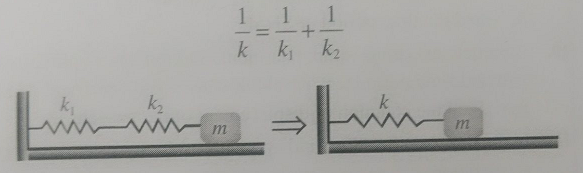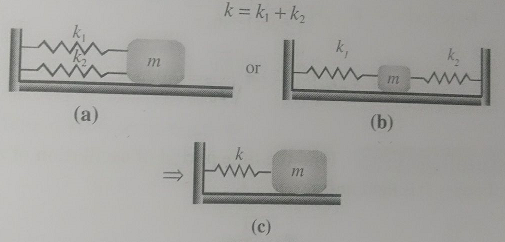# A spring of force constant k is cut into lengths of ratio 1 : 2 : 3. They are connected in series and the new force constant is k. Then they are connected in parallel and force constant is k . Then k' : k" is: Option 1) 1:6 Option 2) 1:9 Option 3) 1:11 Option 4) 1:14

As discussed in

Series combination of spring -- whereinare spring constants of spring 1 & 2 respectively.

and

Parallel combination of spring -- whereinare spring constants of spring 1 & 2 respectively.

Three strings of lengthswith force constantConnected in seriesK' = K

When connected in paralllel

K" = 6K + 3K + 2K = 11KOption 1)

1:6

This option is incorrect

Option 2)

1:9

This option is incorrect

Option 3)

1:11

This option is correct

Option 4)

1:14

This option is incorrect